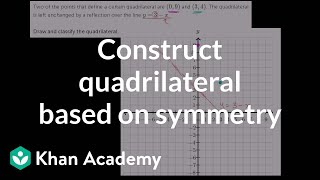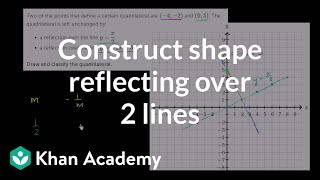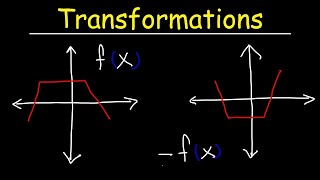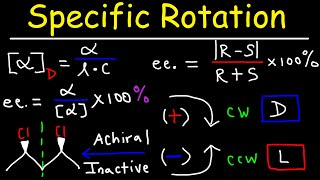# Symmetry of two-dimensional shapes

##By Khan Academy

Sal solves the following problem: Two of the points that define a certain quadrilateral are (0,9) and (3,4). The quadrilateral has reflective symmetry over the line y=3-x. Draw and classify the quadrilateral.# Symmetry of two-dimensional shapes

##By Khan Academy

Sal solves the following problem: Two of the points that define a certain quadrilateral are (-4,-2) and (0,5). The quadrilateral has aï¿½ï¿½ï¿½ï¿½ï¿½ï¿½ï¿½ï¿½ï¿½ï¿½ï¿½ï¿½reflective symmetry over the lines y=x/2 andï¿½ï¿½ï¿½ï¿½ï¿½ï¿½ï¿½ï¿½ï¿½ï¿½ï¿½ï¿½y=-2x + 5.ï¿½ï¿½ï¿½ï¿½ï¿½ï¿½ï¿½ï¿½ï¿½ï¿½ï¿½ï¿½Draw and classify the quadrilateral.# Transformations of Functions

##By The Organic Chemistry Tutor

This precalculus video tutorial provides a basic introduction into transformations of functions. It explains how to identify the parent functions as well as vertical shifts, horizontal shifts, vertical stretching and shrinking, horizontal stretches and compressions, reflection about the x-axis, reflection about the y-axis, reflections about the origins and more. Parent functions include absolute value functions, quadratic functions, cubic functions, and radical functions. This video contains plenty of examples on graphing functions using transformations.# Optical Activity - Specific Rotation & Enantiomeric Excess - Stereochemistry Youtube

##By The Organic Chemistry Tutor

This organic chemistry video tutorial explains how to calculate the specific rotation of an enantiomer given the observed rotation, the pathlength, and the concentration of the solution. It discusses how to determine if a molecule will show optical activity based on its chirality. It also provides the formulas needed to calculate the optical purity and enantiomeric excess. Those formulas can be used to calculate the relative percentages of the R and S isomers.